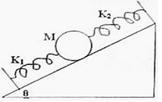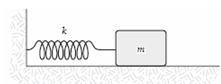Courses

# Test: Examples of Bodies in SHM

## 5 Questions MCQ Test Physics Class 11 | Test: Examples of Bodies in SHM

Description
This mock test of Test: Examples of Bodies in SHM for JEE helps you for every JEE entrance exam. This contains 5 Multiple Choice Questions for JEE Test: Examples of Bodies in SHM (mcq) to study with solutions a complete question bank. The solved questions answers in this Test: Examples of Bodies in SHM quiz give you a good mix of easy questions and tough questions. JEE students definitely take this Test: Examples of Bodies in SHM exercise for a better result in the exam. You can find other Test: Examples of Bodies in SHM extra questions, long questions & short questions for JEE on EduRev as well by searching above.
QUESTION: 1

Solution:
QUESTION: 2

### What provides the restoring force for SHM in case of column of mercury in U-tube?

Solution:

Weight of mercury provides the restoring force for SHM in case of column of mercury in U-tube.

QUESTION: 3

### A sphere of mass M is arranged on a smooth inclined plane of angle Î¸, in between two springs of spring constants K1 and K2 . The springs are joined to rigid supports on the inclined plane and to the sphere (Fig). When the sphere is displaced slightly, it executes simple harmonic motion. What is the period of this motion?Solution:

Compression and expansion in both the springs will be same. Hence, F = k1x= k2x. Fn=F + F. Therefore, kn = k1 + k2.
T = 2π [m / kn]½
T = 2π [m / (k1+k2)]½
Hence A is correct.

QUESTION: 4

The bob of a pendulum of length, I is pulled aside from its equilibrium position through an angle θ and then released. what will be the speed V of the bob when it pass through its equilibrium condition.

Solution:
QUESTION: 5

A mass m = 2.0 kg is attached to a spring having a force constant k = 290 N/m as in the figure. The mass is displaced from its equilibrium position and released. Its frequency of oscillation (in Hz) is approximately:Solution:

We know that T = 2 π√m/k
And f = 1/ T
Thus we get f = 1.9Hz